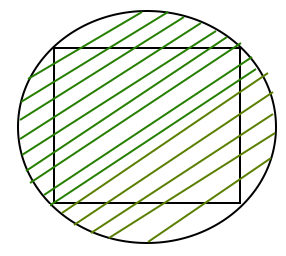Related Articles

# Area of a Circumscribed Circle of a Square

• Difficulty Level : Basic
• Last Updated : 18 Mar, 2021

Given the side of a square then find the area of a Circumscribed circle around it.
Examples:

```Input : a = 6
Output : Area of a circumscribed circle is : 56.55

Input : a = 4
Output : Area of a circumscribed circle is : 25.13```

Attention reader! All those who say programming isn't for kids, just haven't met the right mentors yet. Join the  Demo Class for First Step to Coding Coursespecifically designed for students of class 8 to 12.

The students will get to learn more about the world of programming in these free classes which will definitely help them in making a wise career choice in the future.

All four sides of a square are of equal length and all four angles are 90 degree. The circle is circumscribed on a given square shown by a shaded region in the below diagram.Properties of Circumscribed circle are as follows:

• The center of the circumcircle is the point where the two diagonals of a square meet.
• Circumscribed circle of a square is made through the four vertices of a square.
• The radius of a circumcircle of a square is equal to the radius of a square.

Formula used to calculate the area of inscribed circle is:
(PI * a * a)/2
where, a is the side of a square in which a circle is circumscribed.
How does this formula work?
We know area of circle = PI*r*r.
We also know radius of circle = (square diagonal)/2
Length of diagonal = ?(2*a*a)
Area = PI*r*r = (PI*a*a)/2

## C++

 `// C++ Program to find the``// area of a circumscribed circle``#include ``#define PI 3.14159265` `float` `areacircumscribed(``float` `a)``{``    ``return` `(a * a * (PI / 2));``}` `// Driver code``int` `main()``{``    ``float` `a = 6;``    ``printf``(``" Area of an circumscribed circle is : %.2f "``,``           ``areacircumscribed(a));``    ``return` `0;``}`

## Java

 `// Java program to calculate``// area of a circumscribed circle-square``import` `java.io.*;``class` `Gfg {``    ``// Utility Function``    ``static` `float` `areacircumscribed(``float` `a)``    ``{``        ``float` `PI = ``3``.14159265f;``        ``return` `(a * a * (PI / ``2``));``    ``}` `    ``// Driver Function``    ``public` `static` `void` `main(String arg[])``    ``{``        ``float` `a = ``6``;``        ``System.out.print(``"Area of an circumscribed"``                         ``+ ``"circle is :"``);``        ``System.out.println(areacircumscribed(a));``    ``}``}` `// The code is contributed by Anant Agarwal.`

## Python3

 `# Python3 Program to find the``# area of a circumscribed circle``PI ``=` `3.14159265` `def` `areacircumscribed(a):` `    ``return` `(a ``*` `a ``*` `(PI ``/` `2``))` `# Driver code``a ``=` `6``print``(``" Area of an circumscribed circle is :"``,``        ``round``(areacircumscribed(a), ``2``))``        ` `# This code is contributed by Smitha Dinesh Semwal`

## C#

 `// C# Program to find the``// area of a circumscribed circle``using` `System;` `class` `GFG {``    ` `    ``public` `static` `double` `PI= 3.14159265 ;` `    ``static` `float` `areacircumscribed(``float` `a)``    ``{``        ``return` `(a * a * (``float``)(PI / 2));``    ``}``    ` `    ``// Driver code``    ``public` `static` `void` `Main()``    ``{``        ``float` `a = 6;``        ` `        ``Console.Write(``" Area of an circumscribed"``                            ``+ ``" circle is : {0}"``,``             ``Math.Round(areacircumscribed(a), 2));``    ``}``}` `// This code is contributed by``// Smitha Dinesh Semwal`

## PHP

 ``

## Javascript

 ``

Output :

` Area of an circumscribed circle is : 56.55 `

My Personal Notes arrow_drop_up